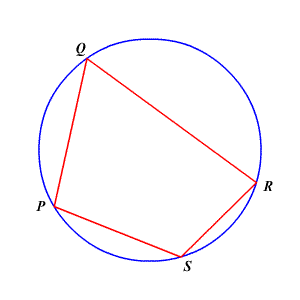# Inscribing a Quadrilateral in a Circle

A quadrilateral can be inscribed in a circle if and only if its opposite angles are supplementary .So, in the image above,

$m\angle PQR+m\angle PSR=180°$

and

$m\angle QPS+m\angle QRS=180°$ .